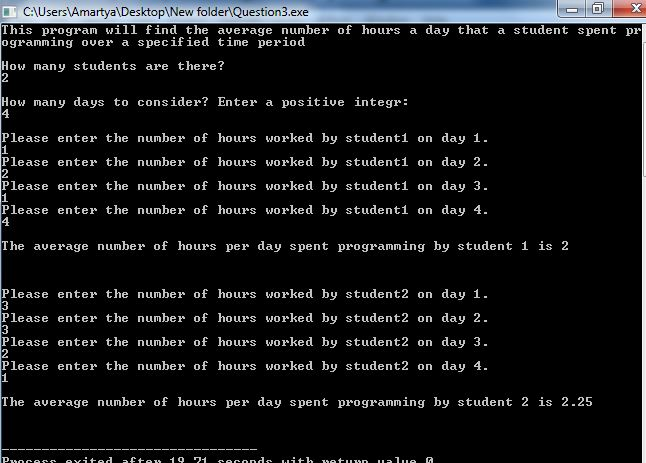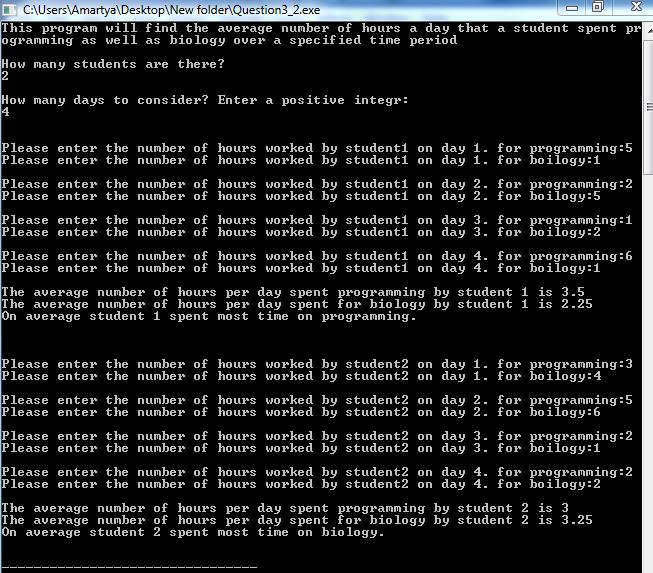# Answered Essay: //Question3.cpp

//Question3.cpp
// This program finds the average time spent programming by a student
// each day over a three day period.

#include<iostream>
using namespace std;

int main()
{
int n;
int numStudents;
float numHours, total, average;
int student, day = 0;   // these are the counters for the loop

cout << “This program will find the average number of hours a day”
<< ” that a student spent programming over a long weekendnn”;
cout << “How many students are there?” << endl << endl;
cin >> numStudents;

for(student = 1; student <= numStudents; student++)
{
total = 0;
for( day = 1; day <= 3; day++)
{
cout << “Please enter the number of hours worked by student”
<< student <<” on day ” << n << “.” << endl;
cin >> numHours;

total = total + numHours;

}
average = total/3;

cout << endl;
cout << “The average number of hours per day spent programming ”
<< “by student ” << student << ” is ” << average
<< endl << endl << endl;
}
return 0;
}

!. The outer loop controls the number of students. Inner loop is always executed three times, once for each day of the long weekend. Modify the code so that the inner loop iterates n times, where n is positive integer that the user inputs.Thus, the users decide how many days to consider just as they choose how many students to consider.

2. Modify so that it also finds the average number of hours per day that a given student studies biology as well as programming. For each given student include two prompts, one for each subject. Have the program display which subject the student, on average, spend most time on.

/*Modified sourcecode for problem#1 is given below:*/

//Question3.cpp

// This program finds the average time spent programming by a student

// each day over a three day period.

#include<iostream>

using namespace std;

int main()

{

int n;

int numStudents;

float numHours, total, average;

int student, day = 0; // these are the counters for the loop

cout << “This program will find the average number of hours a day”

<< ” that a student spent programming over a specified time periodnn”;

cout << “How many students are there?” << endl;

cin >> numStudents;

cout <<endl<< “How many days to consider? Enter a positive integr:”<< endl;

cin >> n;

while(n<=0)

{

cout <<endl<<“Enter correctly!”;

cout <<endl<< “How many days to consider? Enter a positive integr:”<< endl;

cin >> n;

}

for(student = 1; student <= numStudents; student++)

{

total = 0;

cout<<endl;

for( day = 1; day <= n; day++)

{

cout << “Please enter the number of hours worked by student”

<< student <<” on day ” << day << “.” << endl;

cin >> numHours;

total = total + numHours;

}

average = total/n;

cout << endl;

cout << “The average number of hours per day spent programming ”

<< “by student ” << student << ” is ” << average

<< endl << endl << endl;

}

return 0;

}

/*For better understanding output screenshot is attached here*//*Modified sourcecode for problem#2 is given below:*/

#include<iostream>

using namespace std;

int main()

{

int n;

int numStudents;

float numHours, total, average, numHours2, total2, average2;

int student, day = 0; // these are the counters for the loop

cout << “This program will find the average number of hours a day”

<< ” that a student spent programming as well as biology over a specified time periodnn”;

cout << “How many students are there?” << endl;

cin >> numStudents;

cout <<endl<< “How many days to consider? Enter a positive integr:”<< endl;

cin >> n;

while(n<=0)

{

cout <<endl<<“Enter correctly!”;

cout <<endl<< “How many days to consider? Enter a positive integr:”<< endl;

cin >> n;

}

for(student = 1; student <= numStudents; student++)

{

total = 0;

total2 = 0;

cout<<endl;

for( day = 1; day <= n; day++)

{

cout << “nPlease enter the number of hours worked by student”

<< student <<” on day ” << day << “. for programming:”;

cin >> numHours;

cout << “Please enter the number of hours worked by student”

<< student <<” on day ” << day << “. for boilogy:”;

cin >> numHours2;

total = total + numHours;

total2 = total2 + numHours2;

}

average = total/n;

average2 = total2/n;

cout << endl;

cout << “The average number of hours per day spent programming ”

<< “by student ” << student << ” is ” << average

<< endl;

cout << “The average number of hours per day spent for biology ”

<< “by student ” << student << ” is ” << average2

<< endl;

if(average>average2)

cout<<“On average student “<<student<<” spent most time on programming.”<<endl<<endl;

else if(average<average2)

cout<<“On average student “<<student<<” spent most time on biology.”<<endl<<endl;

else

cout<<“On average student “<<student<<” spent same time on biology and programming.”<<endl<<endl;

}

return 0;

}

/*For better understanding output screenshot is attached here*/Calculate your paper price
Pages (550 words)
Approximate price: -

Help Me Write My Essay - Reasons:Best Online Essay Writing Service

We strive to give our customers the best online essay writing experience. We Make sure essays are submitted on time and all the instructions are followed.Our Writers are Experienced and Professional

Our essay writing service is founded on professional writers who are on stand by to help you any time.Free Revision Fo all Essays

Sometimes you may require our writers to add on a point to make your essay as customised as possible, we will give you unlimited times to do this. And we will do it for free.Timely Essay(s)

We understand the frustrations that comes with late essays and our writers are extra careful to not violate this term. Our support team is always engauging our writers to help you have your essay ahead of time.Customised Essays &100% Confidential

Our Online writing Service has zero torelance for plagiarised papers. We have plagiarism checking tool that generate plagiarism reports just to make sure you are satisfied.Our agents are ready to help you around the clock. Please feel free to reach out and enquire about anything.

Try it now!

## Calculate the price of your order

Total price:
\$0.00

How it works?

Follow these simple steps to get your paper doneFill in the order form and provide all details of your assignment.Proceed with the payment

Choose the payment system that suits you most.Receive the final file

Once your paper is ready, we will email it to you.

HOW OUR ONLINE ESSAY WRITING SERVICE WORKS

Let us write that nagging essay.## Submit Your Essay/Homework Instructions

By clicking on the "PLACE ORDER" button, tell us your requires. Be precise for an accurate customised essay. You may also upload any reading materials where applicable.Pick A & Writer

Our ordering form will provide you with a list of writers and their feedbacks. At step 2, its time select a writer. Our online agents are on stand by to help you just in case.Editing (OUR PART)

At this stage, our editor will go through your essay and make sure your writer did meet all the instructions.